# 07 从RDD创建DataFrame

1.pandas df 与 spark df的相互转换

df_s=spark.createDataFrame(df_p)

df_p=df_s.toPandas()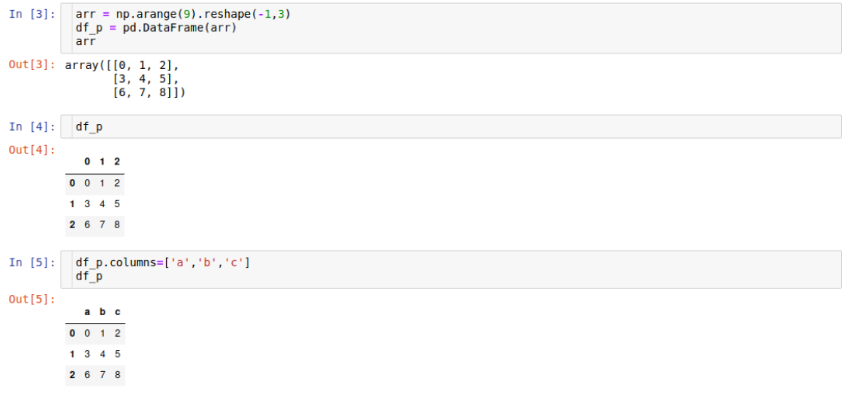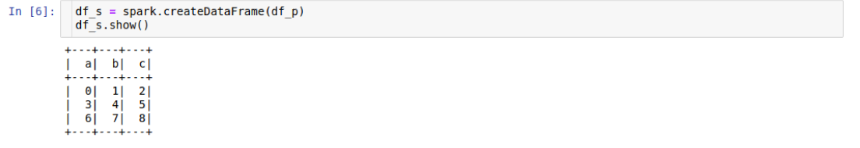2. Spark与Pandas中DataFrame对比

3.1 利用反射机制推断RDD模式

• sc创建RDD
• 转换成Row元素，列名=值
• spark.createDataFrame生成df
• df.show(), df.printSchema()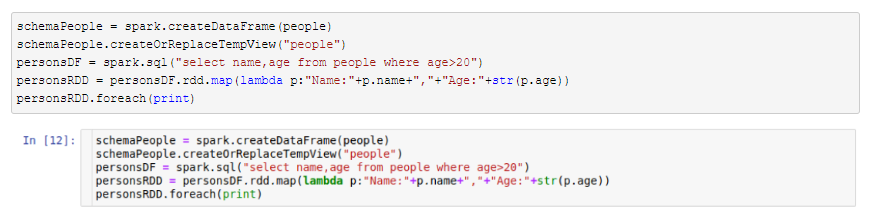3.2 使用编程方式定义RDD模式

• 生成“表头”
• fields = [StructField(field_name, StringType(), True) ,...]
• schema = StructType(fields)
• 生成“表中的记录”
• 创建RDD
• 转换成Row元素，列名=值
• 把“表头”和“表中的记录”拼装在一起
• = spark.createDataFrame(RDD, schema)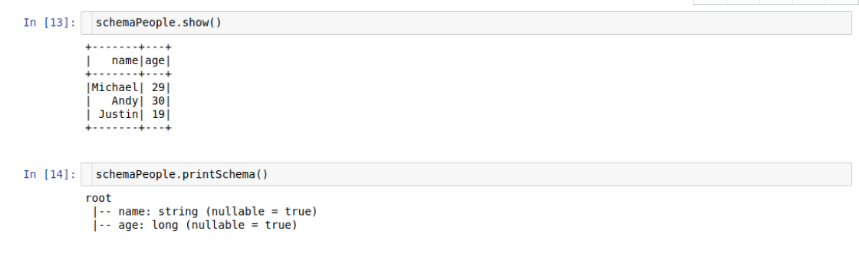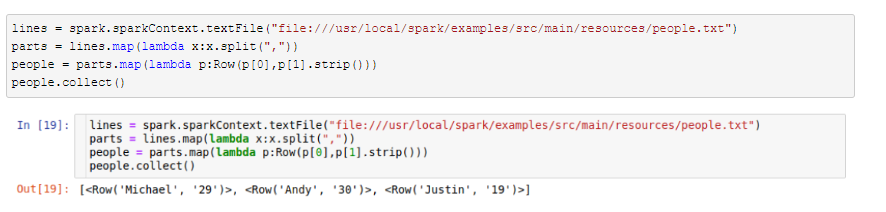4. DataFrame保存为文件

df.write.json(dir)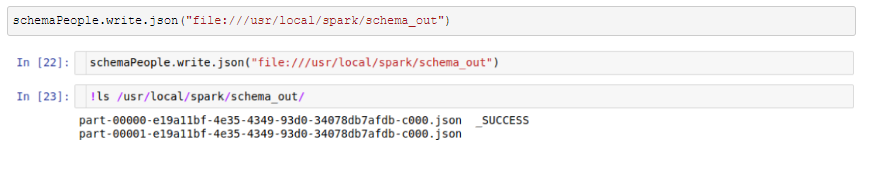posted @ 2021-05-14 21:23  果宝机甲，归位！！！  阅读(34)  评论(0编辑  收藏  举报